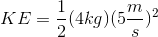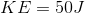# SAT II Physics : Kinetic Energy

## Example Questions

### Example Question #1 : Work, Energy, And Power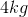cart has a linear momentum with a magnitude of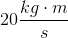. What is the cart's kinetic energy?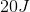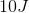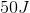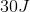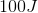Explanation:

Linear momentum is calculated as the product of mass and velocity: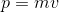We are given the mass of the cart and its momentum, allowing us to solve for its velocity.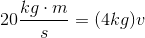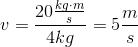Now that we know the velocity of the cart, we need to use the equation for kinetic energy: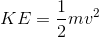Use the value of the velocity and the given mass of the cart to solve.